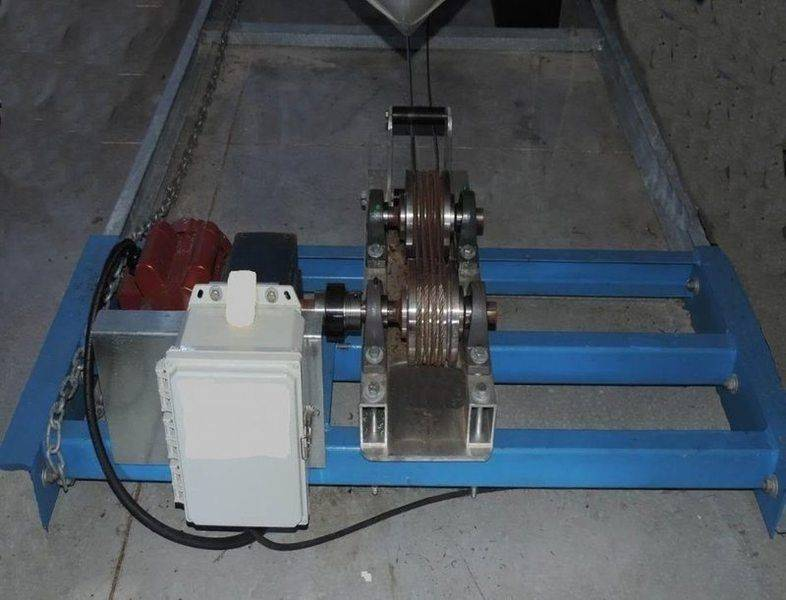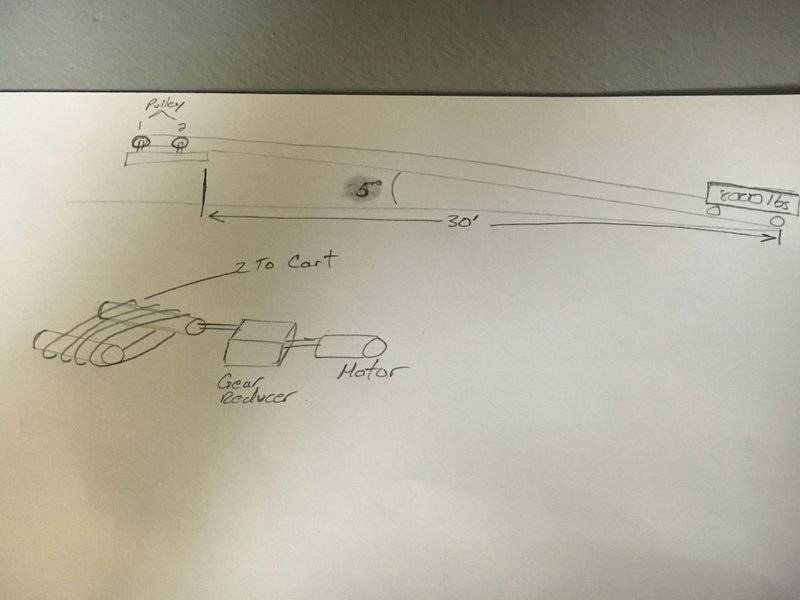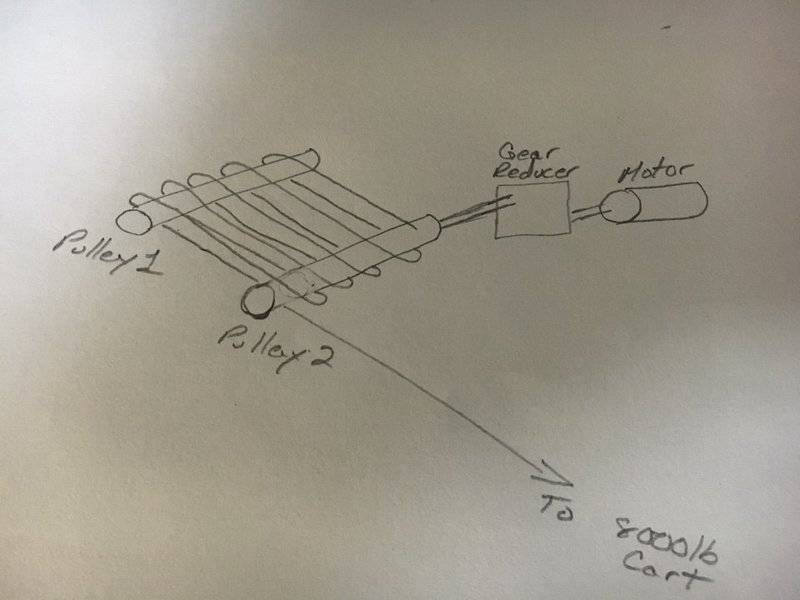# Pulleys, Torque, and inclines

SevenToFive
This was a pulley question that was presented to me today at work. They are using two pulleys, one is driven by a gearbox, the other is sort of an idler, between the two pulleys there is a total of 6 wraps. They are trying to pull a 8000lb cart up and down a straight incline of 5 degrees over 30 feet. The cart is rolling on steel rails.

I am lost on how to calculate the torque needed to pull this cart up their little incline.

Thanks to those who can guide me in the right direction.

How are the six wraps arranged? What is the diameter of the driven pulley? Can you provide a diagram?

benny_91
This was a pulley question that was presented to me today at work. They are using two pulleys, one is driven by a gearbox, the other is sort of an idler, between the two pulleys there is a total of 6 wraps. They are trying to pull a 8000lb cart up and down a straight incline of 5 degrees over 30 feet. The cart is rolling on steel rails.

I am lost on how to calculate the torque needed to pull this cart up their little incline.

Thanks to those who can guide me in the right direction.
It would be much easier to help you if you can post a picture or diagram of your setup with necessary dimensions like the diameter of the pulleys etc.

Gold Member
No matter what, I'm sure it will be more interesting to know the power required than the torque alone.

The pulling force ##F## needed will the part due to gravity (##mg \sin \theta##) and the rolling friction (##f_r mg \cos \theta##), or:
$$F = mg (\sin\theta+f_r\cos\theta)$$
Where:
##mg## = cart weight
##\theta## = slope angle
##f_r## = rolling friction coefficient (0.002 for steel wheels on steel rails)

So putting your numbers in, you need to pull 7132 lb ##= 80 000\times (\sin (5) + 0.002 \cos (5))##.

The power needed is the force times the speed. With the force ##F## in [lb] and the velocity ##v## in [ft/s], you get the power ##P## in [hp]:
$$P = \frac{Fv}{550}$$
For example, if you need to pull the cart 30 feet in 60 s, then your average speed is 0.5 ft/s (= 30 / 60), thus you average power needed to do the work is 6.5 hp (= 7132 X 0.5 / 550). Your motor is required to have that level of power.

For torque & rpm, it is only a matter of ratio. For example, if your motor produces 6.5 hp at 1750 rpm, then it produces 19.5 lb.ft at that rpm (= 5252 X 6.5 / 1750; 5252 is a to keep consistency between units like 550 in the previous equation).

The pulley radius needs to be 0.0027 ft (= 19.5 / 7132) or a diameter of 0.065" to produce the required force and speed. It is of course a ridiculous size, which means you need a mechanical advantage of some sort like gears or pulleys. If your actual pulley diameter is 1.95", then you need to slow down the motion with a pulley ratio of 30:1 (= 1.95 / 0.065) between the shaft pulley and your final pulley where the cable is connected.

SevenToFive
Thanks for the replies everyone. They are currently using 3 inch pulleys but they want to go to 4 inch.Again, thanks for all of the replies.

SevenToFive
Jack Action:
Wouldn't mg be equal to 8000lbs x 32.2ft/s, so 22964.6lbs of force? Which would be the load on the winch correct?

For power the customer is already using a 1HP motor with a 60:1 gear reducer along with two 3" diameter pulleys. These pulleys have 5 wraps around them, which should give some mechanical advantage. How do I calculate the mechanical advantage, and whether or not it can pull up the load?Gold Member
Wouldn't mg be equal to 8000lbs x 32.2ft/s, so 22964.6lbs of force? Which would be the load on the winch correct?
No. 'lb' is already a unit of force. In the US system, we measure a weight in 'lb' and in the SI system we measure a mass in 'kg'. And - of course - weight = mass X g, in either system. The weight in the SI System has the basic unit of Newton and the basic unit for mass in the US system is the slug.

By definition, 1 lbf = 1 slug.ft/s². So the correct way to use ##W = mg## with your numbers is to find the mass in slug which is 8000 lbf / (32.2 ft/s²) = 248.45 slug.

By definition, 1 lbf = 32.2 lbm.ft/s². This means that 1 slug = 32.2 lbm, such that 248.45 slug X 32.2 lbm/slug = 8000 lbm. So you can see that 8000 lbf is equivalent to 8000 lbm under the Earth acceleration. This way, there is no need to do a conversion (at least numerically).

These pulleys have 5 wraps around them, which should give some mechanical advantage. How do I calculate the mechanical advantage, and whether or not it can pull up the load?
As drawn, this configuration doesn't gives a mechanical advantage (if both pulleys are of the same diameter). It only reduces the chance of belt slippage and/or breakage.

So - if I correct the error in my previous post where I used 80 000 lb instead of 8 000 lb - you need 713 lb. With 1 hp, you can pull at speed v = 550 X 1 hp / 713 lb = 0.77 ft/s. It will probably be a little less as there will be some power losses in the transmission components.

The relationship between velocity (in ft/s) and rpm is:
$$v = \frac{rpm \times d}{229.2}$$
Where ##d## is the diameter of the pulley in inches.

So the rpm of 3" pulley must be 58.8 rpm (= 229.2 * 0.77 / 3). Multiply by your mechanical advantage to get the motor rpm and you get 3528 rpm. That should be your motor rpm.

To check, the motor torque should be 1.49 lb.ft (= 5252 * 1 hp / 3528 rpm) or 17.86 lb.in. After the gear reducer, you get 1071.6 lb.in (= 60* 17.86). With the 3" pulley (1.5" radius) you get a pulling force 714 lb (= 1071.6 / 1.5). The error is just due to rounding errors.

3528 rpm sounds about twice as much as a typical electric motor. Either I made a mistake in my conversion, or you have a supplemental mechanical of 2:1 somewhere. From your drawing, it is still not clear how you transform the rotation into linear motion. What is the diameter of the pulley at #2 where the cable winds up?

I assumed it was 3" like the ones with 5 wraps around them. If it was 6", it would reduce the rpm by 2 (1765 rpm). Which implies that the pulley torque is also twice as much.

SevenToFive
What is the diameter of the pulley at #2 where the cable winds up?

I assumed it was 3" like the ones with 5 wraps around them. If it was 6", it would reduce the rpm by 2 (1765 rpm). Which implies that the pulley torque is also twice as much.[/QUOTE]

Correct both pulleys are 3" in diameter, the cable starts on pulley 2 and then goes back and forth to pulley 1 for a total of 5 wraps. At the last wrap of pulley 1 the cable goes to the cart, but rides over pulley 2 not under in my drawing. Thanks for all of your time and effort helping me out with this, it is greatly appreciated.Gold Member
So basically, the two 3" pulleys make a «flat» drum to store the cable as it winds up. In that case, you cannot say you have «5 wraps», as you will have more and more as it winds up (or less and less as it unwinds). The number of wraps is then irrelevant to mechanical advantage and my previous calculations would stand. The key equations are:
At the motor output:
$$P = \frac{T \times rpm}{5252.11}$$
After the gear reducer:
$$T_{out} = T_{in} \times GR$$
$$rpm_{out} = \frac{rpm_{in}} {GR}$$
At the pulley:
$$v = \frac{rpm \times d}{229.183}$$
$$F = \frac{24 T}{d}$$
$$P = \frac{Fv}{550}$$
Where:
• ##P## is power in hp;
• ##T## is torque in lb.ft;
• ##rpm## is angular velocity in rpm;
• ##GR## is the gear ratio of the gear reducer;
• ##v## is the linear velocity of the cable in ft/s;
• ##d## is the diameter of the pulley in inches;
• ##F## is the tension in the cable in lb.
Power in should be equal to power out (I've put more precise constants to make sure of that with the previous equations).

If the tension in the cable is greater than the load it pulls, then the cart will accelerate.

Last edited:
•SevenToFive
SevenToFive
Thank you very much Jack Action for all of your time explaining this to me.